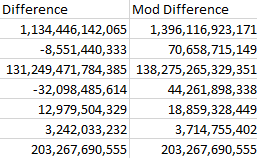# New to Qlik Sense

Discussion board where members can get started with Qlik Sense.

Announcements
Qlik® Product Spotlight: Discover what’s possible. Get more from our products.
See for yourself. Register today.Not applicable

## Arithmetic Expression - Negative to Positive ValuesThe field Difference points to the variable \$(Difference). This variable was defined as the difference between two fields. I am getting this correct. I have defined the field Mod Difference as \$(Difference)*-1 . That is I have multiplied by -1. I am getting different values as result. I am not able to understand why the values are totally different if multiplied by -1. Is any thing wrong with my expression?

I actually wanted to convert values to positive if the difference was negative. Is there any function to do it or do I need to resort to this expression of multiplying by -1?

1 Solution

Accepted Solutions
Honored Contributor II

## Re: Arithmetic Expression - Negative to Positive Values

first

the question is what value you have in your variable because it seems the only part of the sum is multiply by -1

(\$(Difference))*-1 or fabs(\$(Difference))

2 Replies
Honored Contributor II

## Re: Arithmetic Expression - Negative to Positive Values

first

the question is what value you have in your variable because it seems the only part of the sum is multiply by -1